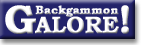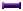TheoryForum Archive : Theory

 Number of no-contact positions

 From: Darse Billings Address: darse@cs.ualberta.ca Date: 23 March 2004 Subject: counting no-contact positions Forum: rec.games.backgammon Google: 1858c7eb.0403232141.1183169e@posting.google.com

```I have counted the exact number of no-contact checker positions
in backgammon (and boy, are my fingers tired:).

The calculation isn't too difficult, but I would like someone
to provide an independent verification.  Specifically, we are
looking for the exact number of no-contact checker positions
with at least one checker of each colour (in other words, all
unfinished pure race positions).

- Darse.
```

 Tom Keith  writes: ```Hi Darse. Let me give it a try. Lemma: n checkers of a given color can be distributed on m consecutive points in (n+m-1)!/(n!(m-1)!) ways. To see why, think of m-1 partitions separating the m points and count the number of ways the m-1 partitions and n checkers can be arranged. Suppose White's highest checker is on the n-point. Then White's remaining 14 checkers are distributed on points 0 thru n. This can happen in (14+n)!/(14!n!) ways. And Black's 15 checkers are distributed on his points 0 thru 24-n. This can happen in (15+24-n)!/(15!(24-n)!) ways. For n=1 to 24, the total number of distrubutions is Sum from n=1 to 24 of (14+n)!/(14!n!) x (15+24-n)!/(15!(24-n)!) = 1402634420740800 There are two other situations to deal with: (n=0) All of White's checkers are off. Then Black's checkers can be on the bar in addition to the 25 other points. That's (15+25)!/(15!25!) ways = 40225345056. (n=25) White has a checker on the bar. Then all of Black's checkers must be off. White's remaining 14 checkers are distributed on points 0 thru 25, in (14+25)!/(14!25!) ways = 15084504396. Adding these up gives: 1402634420740800 40225345056 15084504396 ---------------- 1402689730590252 This includes positions where one side or the other (or both) have all their checkers off. But you said you don't want to include those. If one color has all their checkers off, then the other color's checkers can be distributed in (15+25)!/(15!25!) ways = 40225345056. Subtract this number twice (once for each color having all his checkers off), and add 1 back on so that both colors with all checkers off is not subtracted twice. 1402689730590252 - 40225345056 - 40225345056 + 1 ---------------- 1402609279900141 ```

 Darse Billings  writes: ```Thanks, Tom. That is exactly the number I got. This came up in a dinner conversation with Gerry Tesauro and Michael Buro last week, both of whom have done some excellent work in computer backgammon (Gerry is famous for TD_Gammon, which brought the super strong neural net programs (like Jellyfish and Snowie) into the world). 1.4 quadrillion isn't that big a number any more -- definitely in the feasible range sometime in the future. The Awari oracle (an endgame database that covers every reachable position in the game!) is a little under a trillion positions, and the 10-piece checkers endgame database has more than 39 trillion positions. Building the database would reveal unreachable positions, if there are any. But it isn't clear that it would offer enough value to be worth building, because the equity assessments for race positions can be estimated quite accurately by others means. Here is my solution, and a python script to do the arbitrary precision counting: Define On(n,r) to be the number of ways of putting exactly n checkers on r possibly empty points. This can be done in On(n,r) = choose(n+r-1, r-1) ways. Define Onf(n,r) to be the number of ways of putting n or fewer checkers on r possibly empty points. This is the sum of On(i,r) for i = 0..n. Due to a convenient binomial identity, this is Onf(n,r) = On(n,r+1) = choose(n+r, r). For example, the number of ways of putting 15 or fewer pieces on 6 possibly empty points is equal to the number of ways of putting exactly 15 pieces on 7 possibly empty points. (This makes sense if we imagine that the other 15-i pieces are being placed on the (r+1)th point). I will partition the space into subcases according to the maximum point for the white checker farthest from home. Call this point j (in the range 1..23). Since the maximum point has at least one white checker, the total number of white positions is the sum over i = 1..15 of putting the remaining 15-i or fewer checkers on the lower points, which is Onf(15-i,j-1). Owing to the same binomial identity, this turns out to be equal to Onf(15,j) - Onf(15,j-1). (This makes sense, since the number of ways of leaving the max point empty is equal to the number of ways of putting 15 or fewer checkers over the lower points). The number of corresponding black positions is less constrained, essentially Onf(n,r) for the non-white points, r = 24-j. We need to subtract one from this, because we don't want to include the position with zero black pieces (we want at least one checker of each colour for non-terminal positions). Thus Onf(15,24-j) - 1. The sum of these products is the number of checker positions with white to move (just flip the position if black is to move). A mere 1,402,609,279,900,141 (1.4 quadrillion) positions. The following python script produces the given output (after cleaning it up a bit). (Thanks to Roel van der Goot for the python tips). -=-=- #!/bin/env python def choose(n, r): c = 1L for k in range(min(r, n-r)): c = c * (n-k) / (k+1) return c def on(n, r): # n pieces on r possibly empty points return choose(n+r-1, r-1) def onf(n, r): # n or fewer pieces on r possibly empty points # equals n pieces on r+1 possibly empty points return choose(n+r, r) tp = 0L for j in range(1, 24): pw = onf(15,j) - onf(15,j-1) pb = onf(15,24-j) - 1 pj = pw * pb tp = tp + pj print pj, "=", pw, "*", pb, "split at max point", j print tp, "total no-contact checker positions in backgammon" -=-=- 232069298385 = 15 * 15471286559 split at max point 1 1123703971080 = 120 * 9364199759 split at max point 2 3786173740120 = 680 * 5567902559 split at max point 3 9938706066540 = 3060 * 3247943159 split at max point 4 21581190310932 = 11628 * 1855967519 split at max point 5 40200256444440 = 38760 * 1037158319 split at max point 6 65782237765320 = 116280 * 565722719 split at max point 7 96103737835380 = 319770 * 300540194 split at max point 8 126760485351610 = 817190 * 155117519 split at max point 9 152112581441304 = 1961256 * 77558759 split at max point 10 166894679526600 = 4457400 * 37442159 split at max point 11 167888095064300 = 9657700 * 17383859 split at max point 12 154973615069700 = 20058300 * 7726159 split at max point 13 131131497299400 = 40116600 * 3268759 split at max point 14 101408311376280 = 77558760 * 1307503 split at max point 15 71302628047275 = 145422675 * 490313 split at max point 16 45225023361075 = 265182525 * 170543 split at max point 17 25581509962800 = 471435600 * 54263 split at max point 18 12693999027600 = 818809200 * 15503 split at max point 19 5393905605000 = 1391975640 * 3875 split at max point 20 1890766911000 = 2319959400 * 815 split at max point 21 512500122000 = 3796297200 * 135 split at max point 22 91606302000 = 6107086800 * 15 split at max point 23 1402609279900141 total no-contact checker positions in backgammon ```

### Theory

Derivation of drop points  (Michael J. Zehr, Apr 1998)
Double/take/drop rates  (Gary Wong, June 1999)
Drop rate on initial doubles  (Gary Wong, July 1998)
Error rate--Why count forced moves?  (Ian Shaw+, Apr 2009)
Error rates--Repeated ND errors  (Joe Russell+, July 2009)
Inconsistencies in how EMG equity is calculated  (Jeremy Bagai, Nov 2007)Janowski's formulas  (Joern Thyssen+, Aug 2000)
Janowski's formulas  (Stig Eide, Sept 1999)
Jump Model for money game cube decisions  (Mark Higgins+, Mar 2012)
Number of distinct positions  (Walter Trice, June 1997)
Number of no-contact positions  (Darse Billings+, Mar 2004)
Optimal strategy?  (Gary Wong, July 1998)
Proof that backgammon terminates  (Robert Koca+, May 1994)Solvability of backgammon  (Gary Wong, June 1998)
Undefined equity  (Paul Tanenbaum+, Aug 1997)Under-doubling dice  (Bill Taylor, Dec 1997)Variance reduction  (Oliver Riordan, July 2003)From GammOnLineLong messageRecommended readingRecent addition

 Book Suggestions Books Cheating Chouettes Computer Dice Cube Handling Cube Handling in Races Equipment Etiquette Extreme Gammon Fun and frustration GNU Backgammon History Jellyfish Learning Luck versus Skill Magazines & E-zines Match Archives Match Equities Match Play Match Play at 2-away/2-away Miscellaneous Opening Rolls Pip Counting Play Sites Probability and Statistics Programming Propositions Puzzles Ratings Rollouts Rules Rulings Snowie Software Source Code Strategy--Backgames Strategy--Bearing Off Strategy--Checker play Terminology Theory Tournaments Uncategorized Variations